# Test: Discrete Time Signal Analysis - 2

## 15 Questions MCQ Test Digital Signal Processing | Test: Discrete Time Signal Analysis - 2

Description
Attempt Test: Discrete Time Signal Analysis - 2 | 15 questions in 15 minutes | Mock test for Electrical Engineering (EE) preparation | Free important questions MCQ to study Digital Signal Processing for Electrical Engineering (EE) Exam | Download free PDF with solutions
QUESTION: 1

### Which of the following relation is true if the signal x(n) is real?

Solution:

Explanation: We know that,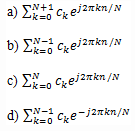QUESTION: 2

### For a signal x(n) to exhibit even symmetry, it should satisfy the condition | X(-ω)|=| X(ω)|.

Solution:

Explanation: We know that, if a signal x(n) is real, then
X*(ω)=X(-ω)
If the signal is even symmetric, then the magnitude on both the sides should be equal.
So, |X*(ω)|=|X(-ω)| =>| X(-ω)|=| X(ω)|.

QUESTION: 3

### What is the energy density spectrum Sxx(ω) of the signal x(n)=anu(n), |a|<1?

Solution:

Explanation: Since |a|<1, the sequence x(n) is absolutely summable, as can be verified by applying the geometric summation formula.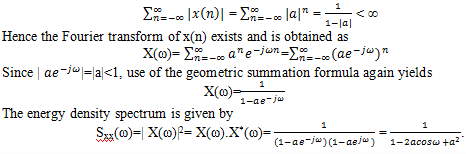QUESTION: 4

What is the Fourier transform of the signal x(n) which is defined as shown in the graph below?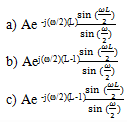None of the mentioned

Solution:

Explanation: The Fourier transform of this signal is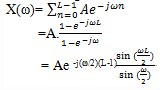QUESTION: 5

Which of the following condition is to be satisfied for the Fourier transform of a sequence to be equal as the Z-transform of the same sequence?

Solution:

Explanation: Let us consider the signal to be x(n)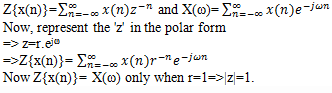QUESTION: 6

The sequence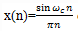does not have both z-transform and Fourier transform.

Solution:

Explanation: The given x(n) do not have Z-transform. But the sequence have finite energy. So, the given sequence x(n) has a Fourier transform.

QUESTION: 7

If x(n) is a stable sequence so that X(z) converges on to a unit circle, then the complex cepstrum signal is defined as:

Solution:

Explanation: Let us consider a sequence x(n) having a z-transform X(z). We assume that x(n) is a stable sequence so that X(z) converges on to the unit circle. The complex cepstrum of the signal x(n) is defined as the sequence cx(n), which is the inverse z-transform of Cx(z), where Cx(z)=ln X(z)
=> cx(z)= X-1(ln X(z))

QUESTION: 8

If cx(n) is the complex cepstrum sequence obtained from the inverse Fourier transform of ln X(ω), then what is the expression for cθ(n)?

Solution:

Explanation: We know that,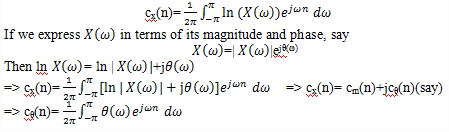QUESTION: 9

What is the Fourier transform of the signal x(n)=u(n)?

Solution:

Explanation: Given x(n)=u(n)
We know that the z-transform of the given signal is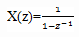ROC:|z|>1
X(z) has a pole p=1 on the unit circle, but converges for |z|>1.
If we evaluate X(z) on the unit circle except at z=1, we obtain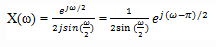QUESTION: 10

If a power signal has its power density spectrum concentrated about zero frequency, the signal is known as:

Solution:

Explanation: We know that, for a low frequency signal, the power signal has its power density spectrum concentrated about zero frequency.

QUESTION: 11

What are the main characteristics of Anti aliasing filter?

Solution:

Explanation: T he anti aliasing filter is an analog filter which has a twofold purpose. First, it ensures that the bandwidth of the signal to be sampled is limited to the desired frequency range. Using an anti aliasing filter is to limit the additive noise spectrum and other interference, which often corrupts the desired signal. Usually, additive noise is wide band and exceeds the bandwidth of the desired signal.

QUESTION: 12

In general, a digital system designer has better control of tolerances in a digital signal processing system than an analog system designer who is designing an equivalent analog system.

Solution:

Explanation: Analog signal processing operations cannot be done very precisely either, since electronic components in analog systems have tolerances and they introduce noise during their operation. In general, a digital system designer has better control of tolerances in a digital signal processing system than an analog system designer who is designing an equivalent analog system.

QUESTION: 13

The term ‘bandwidth’ represents the quantitative measure of a signal.

Solution:

Explanation: In addition to the relatively broad frequency domain classification of signals, it is often desirable to express quantitatively the range of frequencies over which the power or energy density spectrum is concentrated. This quantitative measure is called the ‘bandwidth’ of a signal.

QUESTION: 14

If F1 and F2 are the lower and upper cutoff frequencies of a band pass signal, then what is the condition to be satisfied to call such a band pass signal as narrow band signal?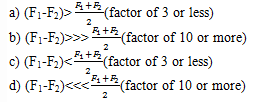Solution:

Explanation: If the difference in the cutoff frequencies is much less than the mean frequency, the such a band pass signal is known as narrow band signal.

QUESTION: 15

What is the frequency range(in Hz) of Electroencephalogram(EEG)?

Solution:

Explanation: Electroencephalogram(EEG) signal has a frequency range of 0-100 Hz.Use Code STAYHOME200 and get INR 200 additional OFF Use Coupon Code